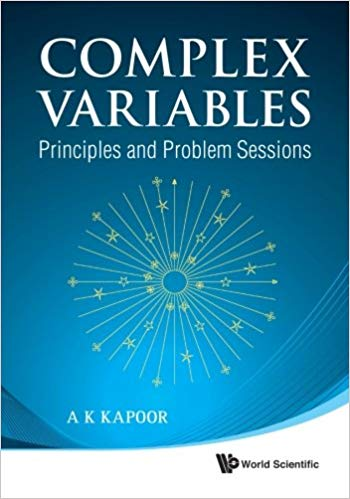# PSN/CV-05001 Taylor series for $z^q(z-1)^p$

For page specific messages
For page author info

Category:

 Problem Find the first three terms of the Taylor series of the function $$f(z)=z^p (z-1)^q$$about  the point $$z_0=-2$$. Assume the branch cuts for the functions $$z^p$$ and $$(z-1)^q$$ along the positive real axis. Write the answer for the special case of $$p+q=1$$

### Session Objective

In this problem solving session you will learn how to obtain Taylor expansion of a function with branch points.

### Prerequisites

A good general understanding of functions with branch point and branch cuts is a must for this session.
If you are not sure of your understanding of  functions with branch cut and branch points, it is good
idea to make a beginning now. A good starting point is Chapter 3 
Important: Several concepts and results about functions with branch points will be used here without any warning.

### Skills required

• How to define  function  $$(z-a)^\lambda$$ when branch cut is given
• How to use definition and  compute values of the functions;
• How to obtain Taylor Series by finding successive derivatives;

### Quick Reminder

The Taylor series of a function $$f(z)$$ in powers $$(z-z_0)$$ exists if
the given function is analytic  at $$z_0$$ and is given by \begin{equation}  f(z) =\sum_{n=0}^\infty a_n (z-z_0)^n,
\end{equation} where \begin{equation}  a_n= \frac{1}{n!}\frac{d^n f(z)}{dz^n}\Big|_{z=z_0} \end{equation}Download cv-ymp-05001.pdf (51.85 KB)### Exclude node summary :

n

Reference:
 A. K. Kapoor,  Complex Variables -- Principles and Problem Sessions,
World Scientific Singapore (2011).

Document Type :: Problem Solving Session     Page-Id :: PSN/CV-05001   File-Id cv-psn-05001.pdf

## Complex Variables :: Solutions and Errata

Complex Variables --- Principles and Problem Sessions  SOLUTIONS and ERRATA

### Chapter 4 :: Integration in Complex Plane

• $$\S\S$$ 4.1 Questions Range of Parameters In Improper Integrals
• $$\S\S$$ 4.2 Tutorial Computing Line Integrals in Complex Plane
• $$\S\S$$ 4.3 Exercise Evaluation of Line Integrals
• $$\S\S$$ 4.4 Questions Deformation of Contours
• $$\S\S$$ 4.5 Exercise Deformation of Contours
• $$\S\S$$  4.6 Exercise Cauchy Theorem
• $$\S\S$$ 4.7 Tutorial Shift of Real Integration Parameter by a Complex Number
• Q Prove that $$\int_0^\infty exp(-px^2) \cos(2bx)\, dx= \frac{1}{2}\sqrt{\frac{\pi}{p}} \exp(-b^2/p), p>0$$
• Q Prove that $$\int_0^\infty e^{-px^2} \sin(ax) \sin(bx) = \frac{1}{4} \left\{\exp\Big(\frac{(a-b)^2}{4p}\Big)- \exp\Big( -\frac{-(a+b)^2}{4p}\Big) \right\}$$
• $$\S\S$$ 4.8 Tutorial Scaling of a Real Integration Variable by a Complex Number
• $$\S\S$$ 4.9 Exercise Shift and Scaling by a Complex Number
• $$\S\S$$ 4.10 Exercise Rotation of Contour
• $$\S\S$$  4.11 Mixed Bag

### Chapter 5 :: Cauchy Integral Formula

• $$\S\S$$ 5.2
• $$\S\S$$ 5.3
• $$\S\S$$ 5.5
• $$\S\S$$ 5.4 :: Taylor Series Representation
• $$\S\S$$ 5.8 :: Laurent Expansion of $$f(z)$$ in powers of $$(z-z_0) ### Chapter 6 :: The Residue Theorem ### Chapter 7 :: Contour Integration • \(\S\S$$ 7.2 Tutorial Integrals of Rational Function
• $$\S\S$$ 7.3 Exercise
• $$\S\S$$ 7.4 Exercise ---Integrals of $$\sin x / \cos x$$ with rational functions.
• $$\S\S$$ 7.5 Tutorial Integration Around a Branch Cut
• $$\S\S$$ 7.6 Integration around a branch cut
• $$\S\S$$ 7.7 Integral of Type $$\int • \(\S\S$$ 7.8 Hyperbolic Functions
• $$\S\S$$7.9 Exercise ----- Exponential and Hyperbolic Functions
• $$\S\S$$ 7.10 Tutorial Principal Value Integrals
• $$\S\S$$ 7.11 Exercise Integrals Requiring Indented Contours
• $$\S\S$$ 7.12 Series Summation and Expansion
• $$\S\S$$ 7.13 What you see is not what you get
• $$\S\S$$ 7.14 Integrals from Statistical Mechanics
• $$\S\S$$ 7.15 Alternate Routes Improper Integrals
• $$\S\S$$7.16 Killing Two Birds with One Stone
• $$\S\S$$ 7.17
• $$\S\S$$ 7.18 Mixed Bag : Improper Integrals

### Errata for Complex Variables ----Principles and Problem Sessions

This Collection has
(a) Solutions for Problem Sessions and
(b) Errata
for my

PLEASE EXPAND THE TABLE OF CONTENT AND CLICK ON SECTION OF INTEREST

I take this opportunity to thank my friend and colleague, Prof. T. Amarnath, from School of Mathematics, University of Hyderabad for his kind words of appreciation and for recommending the book to National Board of Higher Mathematics for inclusion in scheme of distribution of mathematics books to Universities and Institutes in India.Starting with this page the solutions to the problem sessions in the book Complex Variables --- Principles and Prolems Sessions published by World Scientific Singapore (2011) are being made available for reference
n